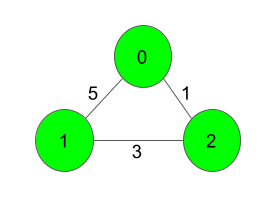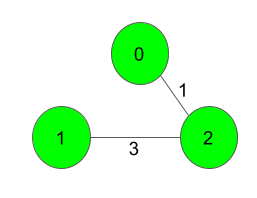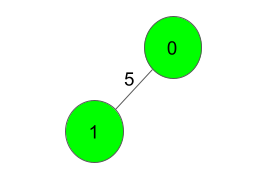XDAYS

:

HOUR

:

MINS

:

SEC

Copied to Clipboard
Minimum Spanning Tree
Medium Accuracy: 49.39% Submissions: 51569 Points: 4

Given a weighted, undirected and connected graph of V vertices and E edges. The task is to find the sum of weights of the edges of the Minimum Spanning Tree.

Example 1:

Input:Output:
4
Explanation:The Spanning Tree resulting in a weight
of 4 is shown above.


Example 2:

Input:Output:
5
Explanation:
Only one Spanning Tree is possible
which has a weight of 5.


Since this is a functional problem you don't have to worry about input, you just have to complete the function  spanningTree() which takes number of vertices V and an adjacency matrix adj as input parameters and returns an integer denoting the sum of weights of the edges of the Minimum Spanning Tree. Here adj[i] contains a list of lists containing two integers where the first integer a[i] denotes that there is an edge between i and a[i] and second integer a[i] denotes that the distance between edge i and a[i] is a[i].

Expected Time Complexity: O(ElogV).
Expected Auxiliary Space: O(V2).

Constraints:
2
V 1000
V-1
E (V*(V-1))/2
1
w 1000
Graph is connected and doesn't contain self loops & multiple edges.

### Editorial

We strongly recommend solving this problem on your own before viewing its editorial. Do you still want to view the editorial?

#### My Submissions: